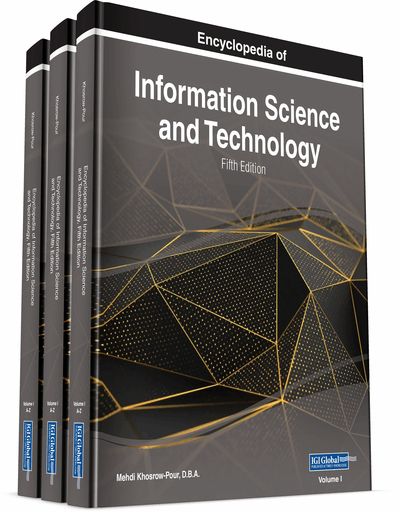# Convolutional Neural Network

Mário Pereira Véstias
DOI: 10.4018/978-1-7998-3479-3.ch002
OnDemand:
(Individual Chapters)
Available
\$37.50
No Current Special Offers

## Abstract

Machine learning is the study of algorithms and models for computing systems to do tasks based on pattern identification and inference. When it is difficult or infeasible to develop an algorithm to do a particular task, machine learning algorithms can provide an output based on previous training data. A well-known machine learning model is deep learning. The most recent deep learning models are based on artificial neural networks (ANN). There exist several types of artificial neural networks including the feedforward neural network, the Kohonen self-organizing neural network, the recurrent neural network, the convolutional neural network, the modular neural network, among others. This article focuses on convolutional neural networks with a description of the model, the training and inference processes and its applicability. It will also give an overview of the most used CNN models and what to expect from the next generation of CNN models.
Chapter Preview
Top

## Introduction

In a broad sense, a convolutional neural network is one of many methods to achieve artificial intelligence.

Artificial intelligence (AI) is a field of computer science dedicated to the research of methods and algorithms that permit to perceive information from the environment, learn from it and taking actions and decisions based on the learning outcomes without any explicit orientation from external agents. AI consists of many sub-fields, including evolutionary computation (e.g., genetic algorithms), vision (e.g. object recognition), expert systems (e.g. decision support systems), speech processing (e.g. speech recognition), natural language processing (e.g., machine translation), and machine learning (e.g. decision trees).

Machine learning (ML) is a type of algorithms and models for computing systems to do tasks based on pattern identification and inference (Mitchell, T., 2017). When it is difficult or infeasible to develop an algorithm to do a particular task, machine learning algorithms can provide an output based on previous data. Machine learning algorithms identify features from data and build models from these features so that new decisions can be produced based on these models and rules. Examples of machine learning models include deep learning, support vector machines (SVM), genetic algorithms, clustering, dimensionality reduction, artificial neural network, decision tree and others.

In this chapter we are particularly interested in deep learning (DL) (Aggarwal, C., 2018). Deep learning teach computers to learn by example. Deep learning first appeared in 1980, but only recently has been applied in practice because it requires large amounts of labeled data and high-performance computing. Its application is vast including areas like computer vision, speech recognition, language processing, among others.

The most recent deep learning models are based on artificial neural networks (ANN) with a large number of layers. Deep learning networks are first trained using a large set of labeled data in order to learn features from the training data without being explicitly programmed to learn them.

There are several types of artificial neural networks including the feedforward neural network, the Kohonen self organizing neural network, the recurrent neural network, the convolutional neural network, the modular neural network, among others. The convolutional neural network (CNN) is one of the most used deep learning architecture for artificial intelligence (see figure 1).

Convolutional neural networks are one of the most used deep learning models in the industry and business. Therefore, for those interested in understanding how deep learning is applied in this markets it is fundamental to understand the architecture of CNNs and understand the evolution and trends of CNNs during the last decade.

This article focus on convolutional neural networks with a description of the model, the training and inference processes and its applicability. It will also give an overview of the most used CNN models and what to expect from the next generation of CNN models.

Top

## Background

Artificial neural network (ANN) is a machine learning model that mimics the structure of the human brain consisting of interconnected neurons. Theoretically, an ANN is a universal model capable to learn any function (Hornik at el., 1989). A class of ANN is the convolutional neural network introduced in (LeCun, Y. et al., 1989).

Convolutional neural networks process images. The network applies successive convolutions to the input image using several trained filters to identify features of the image. Features identified in a convolution are process by the next convolutions to identify more complex features. The process permits to learn complex features and at the same time is invariant to translation of the features.

## Key Terms in this Chapter

Softmax Function: A function that takes as input a vector of k values and normalizes it into a probability distribution of k probabilities.

Pooling Layer: A network layer that determines the average pooling or max pooling of a window of neurons. The pooling layer subsamples the input feature maps to achieve translation invariance and reduce over-fitting.

Convolutional Layer: A network layer that applies a series of convolutions to a block of input feature maps.

Fully Connected Layer: A network layer where all neurons of the layer are connected to all neurons of the previous layer.

Artificial Neural Network (ANN): It is a computing model based on the structure of the human brain with many interconnected processing nodes that model input-output relationships. The model is organized in layers of nodes that interconnect to each other.

Convolutional Neural Network (CNN): A class of deep neural networks applied to image processing where some of the layers apply convolutions to input data.

Unsupervised Training: A training process of neural networks where the training set does not have the associated outputs.

Deep Neural Network (DNN): An artificial neural network with multiple hidden layers.

Feature Map: A feature map is a 2D matrix of neurons. A convolutional layer receives a block of input feature maps and generates a block of output feature maps.

Deep Learning (DL): A class of machine learning algorithms for automation of predictive analytics.

## Complete Chapter List

Search this Book:
Reset